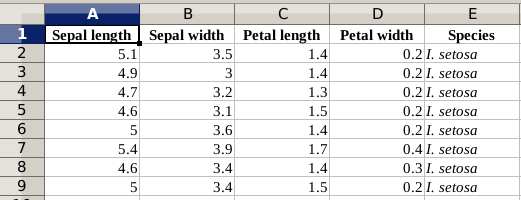Python hosting: Host, run, and code Python in the cloud!

Related course:
Data Analysis with Python Pandas

We import the pandas module, including ExcelFile. The method read_excel() reads the data into a Pandas Data Frame, where the first parameter is the filename and the second parameter is the sheet.

The list of columns will be called df.columns.

Using the data frame, we can get all the rows below an entire column as a list. To get such a list, simply use the column header

We start with a simple Excel file, a subset of the Iris dataset.To iterate over the list we can use a loop:

We can save an entire column into a list:

We can simply take entire columns from an excel sheet:

Related course:
Data Analysis with Python Pandas